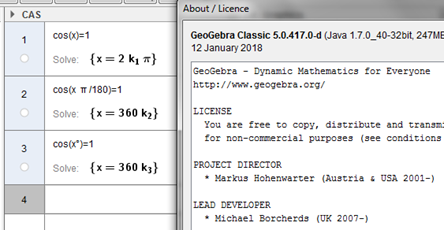# cos(x°)=1 gives x = 3330 in CAS

mrahikka shared this problem 2 years ago
Not a Problem

If we solve (in CAS)

cos(x°)=1

and click solve numercally, we get x = 3330 in GeoGebra 5 and GeoGebra 6.

GeoGebra Graphing Calculator gives Undefined as a solution.

I guess this should be fixed.1

what version?1

The equation is cos(x°) = 0, and you should click on Solve numerically button.

Sorry, my mistake on equation.

M

Files: cos.png1

I think the help is enough explicit (cos(x°)=0,x=100) or (cos(x°)=0,x=200)

```Note:

If you don't give a starting point like a=3 or {x = 3, y = 1.5} the numerical algorithm may find it hard to find a solution (and giving a starting point doesn't guarantee that a solution will be found)
The number of decimals depends on the choosen in global rounding.
NSolve won't work for functions that are asymptotic to the x-axis or other extreme examples. They can often be reformulated though.```